# Gamma

Gamma is the rate of change in delta (∆) with respect to changes in the underlying price of a security.

## The Best Brokers For Gamma-Based Trading Strategies

#1
Forex.com
Bonus
Active Trader Program With A 15% Reduction In Costs
#2
eToro USA
Bonus
Invest \$100 and get \$10
eToro USA LLC and eToro USA Securities Inc.; Investing involves risk, including loss of principal; Not a recommendation
#3

Gamma is known as what’s called a second-order Greek. It’s the second derivative of the value function (i.e., value of an option) with respect to an asset’s underlying price (S).

Gamma = ∂∆ / ∂S = ∂^2V / ∂S

Being “long gamma” generally means being long options. Being “short gamma” generally means being short options.

When long options increase in value, delta increases as well. The rate of change that that delta leads to the concept of gamma.

Gamma increases as an option nears the at-the-money (ATM) point and declines the further out-of-the-money (OTM) or in-the-money (ITM) it is.

##### Call Gamma (blue) vs. Delta (red)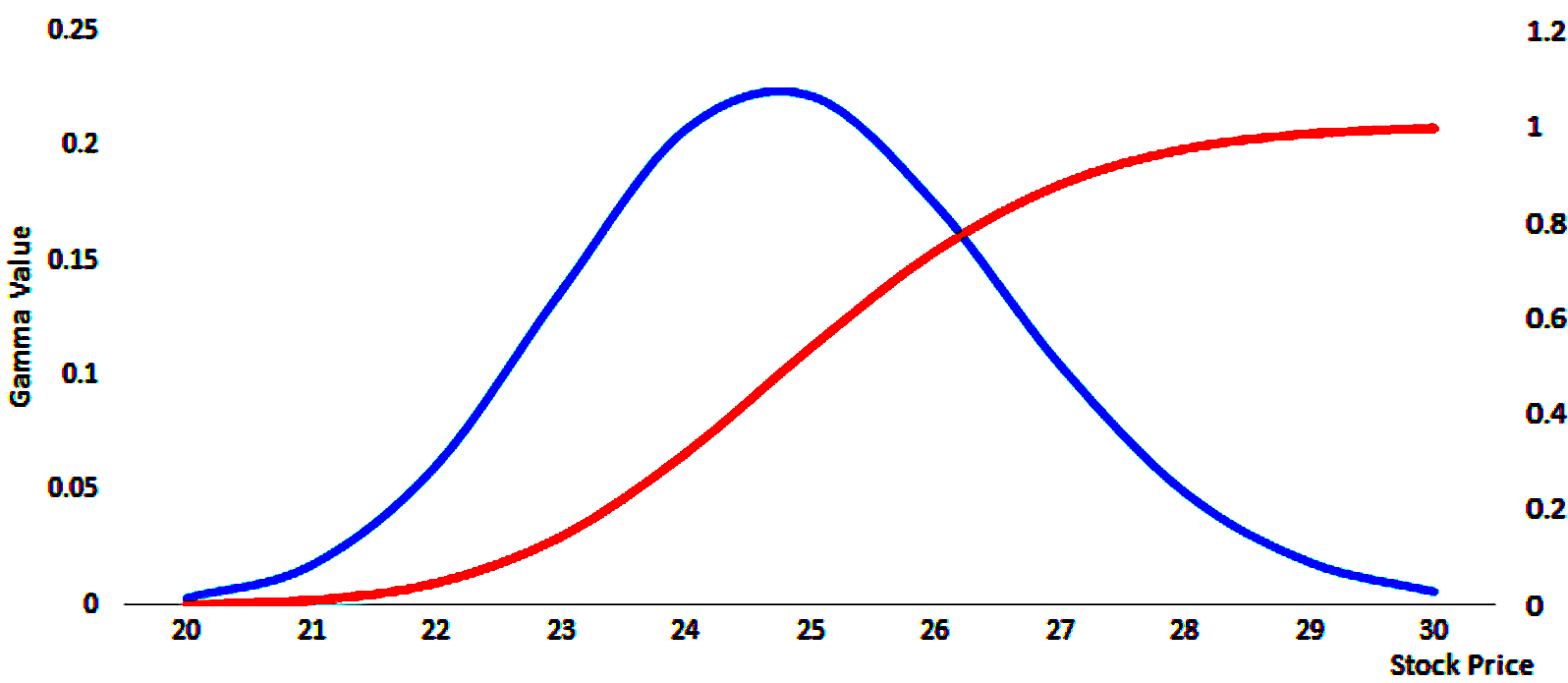As gamma increases, delta goes from 0 to about 0.50 for long call options; and from 0 to about minus-0.50 for long put options.

Delta (red) looks like a logistic growth function. Its rate of change (gamma) is highest in the center before inflecting and the rate of change becomes lower, though still positive.

This information is reflected in the blue curve. It shows that when an option is ATM and begins to go ITM, an option begins to act more like the underlying asset. Delta’s rate of change thereby decreases, which causes gamma to decrease.

Gamma is lowest for deltas of 0.0 and 1.0 for call options and lowest for delta of 0.0 and minus-1.0 for put options.

(The inverse is true for short call and put options.)

## Exceptions

It is generally understood that “long gamma” involves being long options and “short gamma” involves being short options.

The exception to this is when there is negative convexity in the payout function.

Convexity is normally positive when being long options.

This is because you pay a fixed premium for the option, and if you get a favorable move, delta increases non-linearly (positive gamma). That gives options a type of leverage or a type of convexity in the potential payoff structure.

Negative convexity is rare. But it does exist in some circumstances and in some parts of the financial markets.

When convexity is negative that means the shape of a bond’s yield curve is concave.

For example, most mortgage-backed securities (MBS) – i.e., bonds that are tied to mortgage lending and subsequent servicing of that – exhibit negative convexity.

This is because of refinancing risk.

In most mortgages, a homeowner takes out a fixed-rate loan. If interest rates fall below the rate they have on their mortgage, many will try to refinance their mortgage.

This helps lock in lower monthly payments and is favorable for the borrower.

But for the investors in mortgage-backed securities, it’s riskier because they are holding onto a bond that’s likely to be paid off faster than what they initially believed.

When interest rates fall, the prices of these mortgage-backed securities rise less than other bonds of similar maturities.

This is due to the fact the amount of time that’s expected to elapse between when the security was issued and when it matures has decreased.

Consequently, most mortgage bonds exhibit negative convexity. Derivatives tied to them may also pose a similar type of relationship due to the inherent concavity in the underlying.

Traders know that gamma is highest approximately ATM and gets lower the further an option is OTM or ITM.

Since gamma is essentially the convexity of an option’s value, traders will think of it from the perspective of risk/reward.

Shorting OTM options can lead to losses in a non-linear way if left uncovered, so traders need to either avoid such exposures or be adept at hedging them.

This is especially important when option expiration is close. Options values erode with time (theta).

OTM options near expiration can change significantly in value even if there’s an otherwise normal move in the underlying.

For some traders it might be compelling at the end of a week, just before options expiration in many securities, to try and squeeze out some extra P/L by shorting options very near expiration. But it can backfire when a small move in the underlying can lead to a loss of multiples of the premium.

Delta hedging works on small price movements. But because of the convexity of an option’s value, it doesn’t do a good job of protecting exposures as it pertains to larger swings in movements of the underlying.

This is where gamma hedging comes in.

An effective delta hedge for a portfolio works on only a small range of price movements in the underlying, so a trader may also try to neutralize the portfolio’s gamma.

This helps ensure that the portfolio is hedged across a wider variety of price movements in the underlying should they occur rapidly. This could be due to a data release – e.g., macroeconomic data, earnings – or anything that changes the discounted set of expectations that drives the price.

## Delta Hedging vs. Gamma Hedging

Delta is the first derivative of the profit and loss curve of the underlying. Gamma is the second derivative of the profit and loss curve.

Hedging involves offsetting adverse exposure in these regards by using a combination of the underlying asset, derivatives, and other exposures to get the desired delta or gamma, whether that’s zero, positive, or negative, depending on what the trader is trying to do.

### Delta Hedge Example

A basic delta hedge could be shorting call options and going long a certain number of shares of the underlying based on the delta of the options and the overall exposure from the options.

That looks like the following. You have that linear delta-1 exposure, but cap it off at a certain price under the standard covered call structure. Also, if the underlying decreases in price, some of that loss is offset by the options premium received.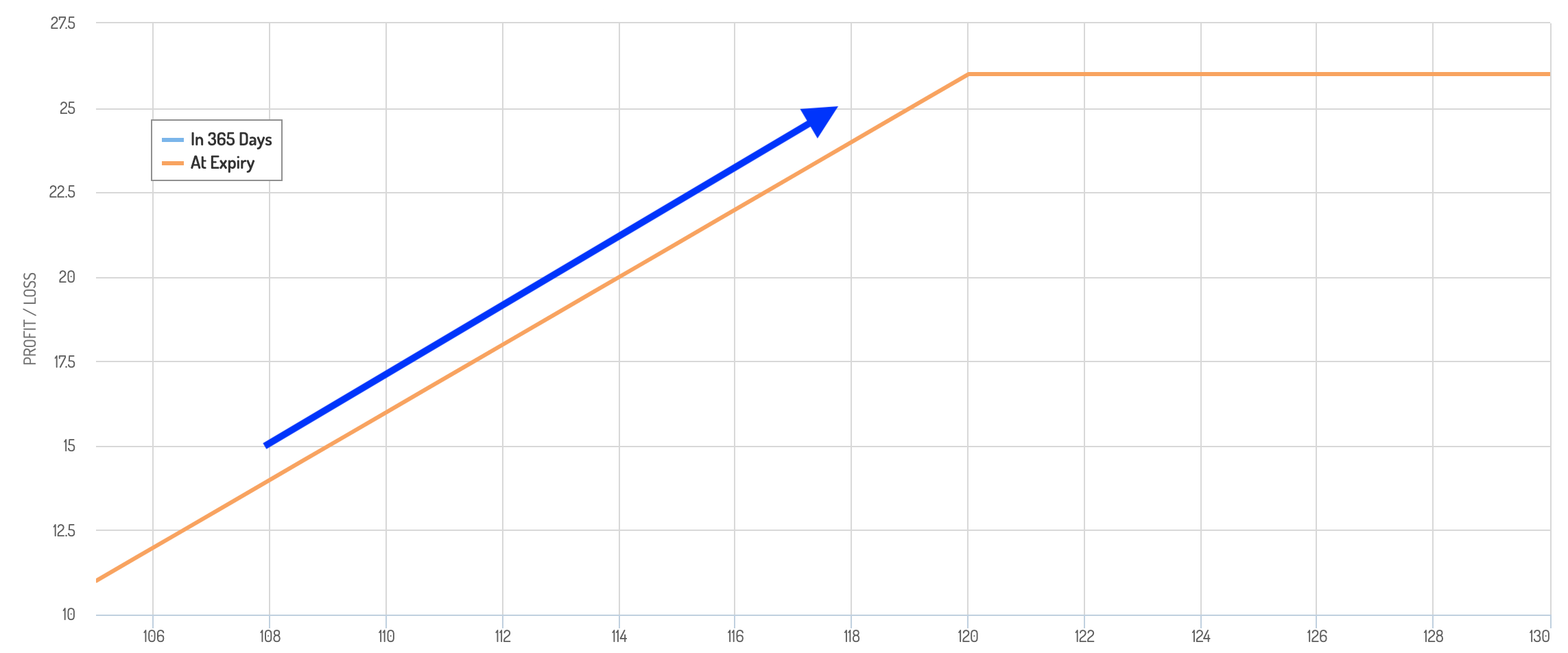__

Alternatively, one could own shares of a stock and go long put options to help neutralize adverse left-tail exposure.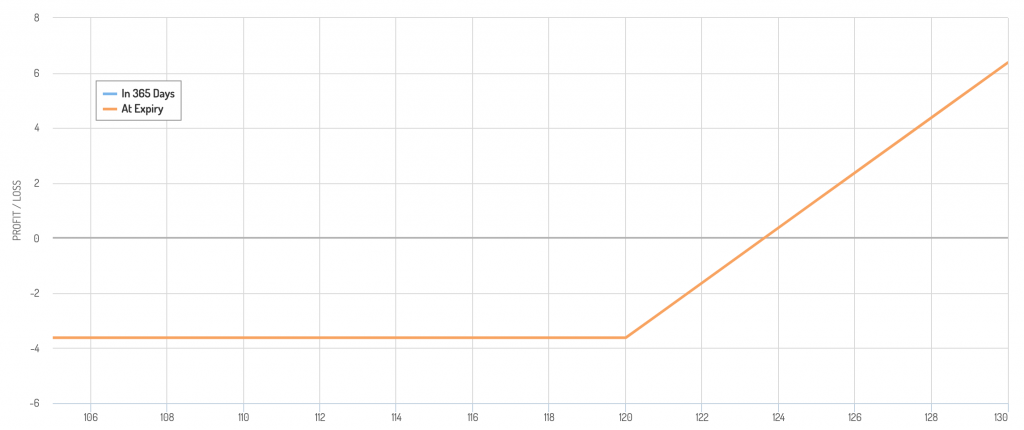### Gamma Hedging

We’ll go through a couple examples.

#### Example #1

An example of a gamma hedge is when someone buys a call option, which gives positive delta and positive gamma, then sells a put to hedge out the gamma but give even more positive delta. (If that put goes ITM, you agree to add long exposure to the underlying asset.)

In terms of a payoff diagram, let’s say the underlying is 115, you buy a call with a 120 strike and sell a put with a 110 strike.

You have a “dead spot” in the 110 to 120 range, where your P/L is fixed. It moves higher above 120 due to the call and falls lower below 110 because of the short put when you effectively start to become short the underlying.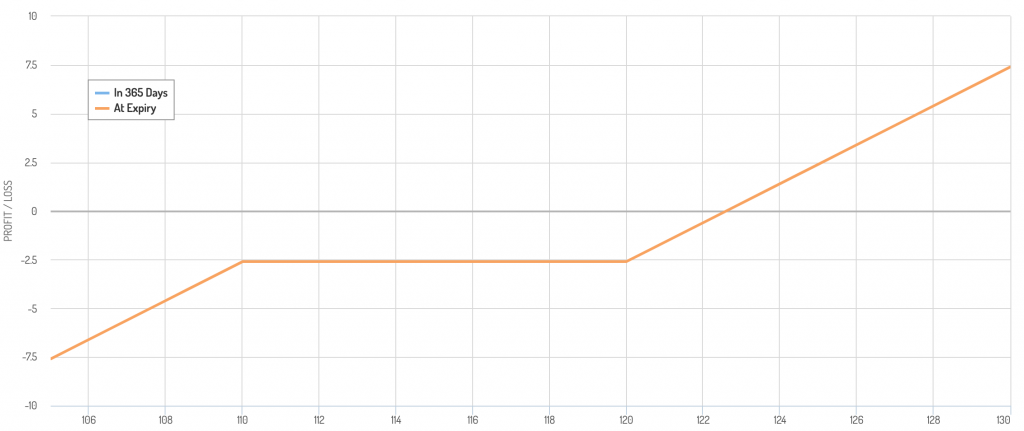Because of your concentrated delta exposure, you would be exposed to large movements in the underlying. So, you could want to short a certain number of shares to help reduce that risk.

#### Example #2

Another example of gamma hedging could come in the form of a bullish collar turned into a condor-like structure.

The setup:

1) Long stock at 115

2) Long put option at 110 (i.e., delta neutralized below 110)

3) Short call option at 120 (i.e., position covered and delta neutralized)

4) Short call option at 130 (i.e., effectively short)

It looks like so: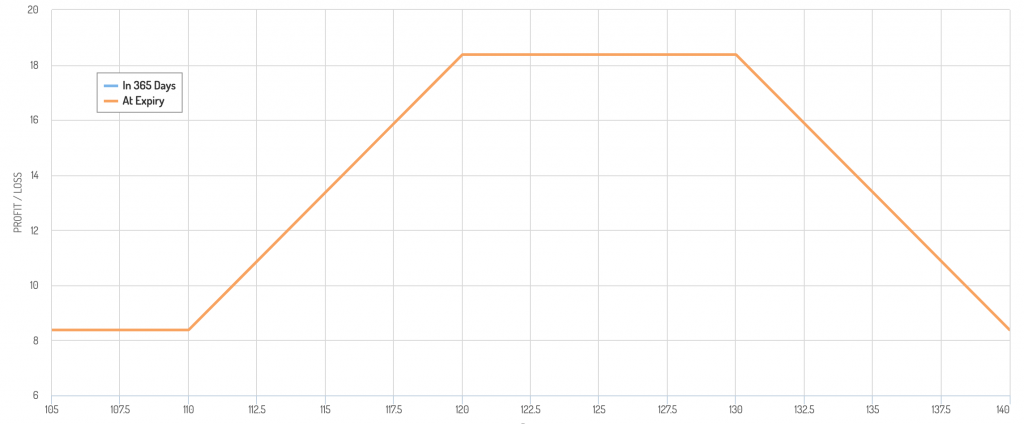Shorting an additional OTM call is a type of gamma hedge by offsetting fluctuations in gamma over a wider range in movements in the underlying.

The trade-off is that you shift more risk back into delta, as it shows in the downward slanting P/L as price goes above 130.

Given price is at 115, going up to 130 is generally a lower-probability event relative to closer prices, but gamma hedging can present tail risks elsewhere.

A similar type of gamma hedge as part of this trade would be to short a put at 100. That would provide additional premium but create adverse delta exposure below 100.

Like delta hedging as it pertains to delta, gamma hedging only protects against smaller movements in gamma.

This is because options have non-zero “speed” (also known as DgammaDspot or “gamma of the gamma”), which relates to the third derivative of the profit and loss curve of the underlying.

Graphically, this relationship of the first, second, and third derivatives of an option’s value with respect to the value of the underlying  is shown below:

##### Delta (blue) vs. Gamma (green) vs. Speed (orange)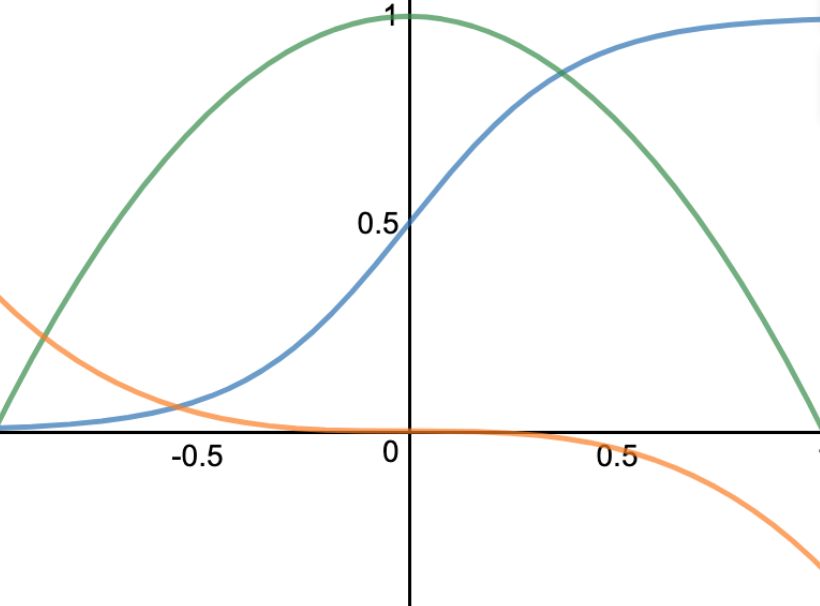## Hedging One Thing May Bring Unintended Exposures In Other Things

As you hedge higher-order derivatives, you can also begin to exposure yourself to changes in other sensitivities, such volatility and time and their higher-order derivatives.

In the gamma hedging examples above, doing so neutralized a second-order effect and ended up giving you a more concentrated delta (a potentially adverse first-order effect).

It’s a natural tendency to overweight first-order effects and pay less attention to the subsequent-order effects because you don’t see them or don’t think they’re that important.

But it can create surprise, unintended exposures.

## Hedging Is About Lowering Exposure, But Not Necessarily Eliminating It

It’s also important to note that oftentimes a trader will seek a hedge that is delta or gamma neutral, but not always.

A trader may also want to have a specific delta position based on what they think about the underlying.

Are they bullish or bearish? The answer to that could determine whether they want a positive or negative delta (i.e., bullish or bearish exposure to the underlying).

That delta could be positive or negative and gamma could be neutral at the same time.

Moreover, traders often want a positive gamma. It can give them convex P/L outcomes for any given move in the underlying.

At the same time, some traders may actually want a negative gamma. Negative gamma means being short options, which can mean a flow of income from selling the premium.

For example, if a stock is trading at \$115 and a trader wants to buy it should it dip to \$100, they might short a put option with a 100 strike in the quantity desired, if possible.

That represents a short gamma position. But it could be something they’d like to do for purposes of both income generation and buying a security that could potentially be on sale.